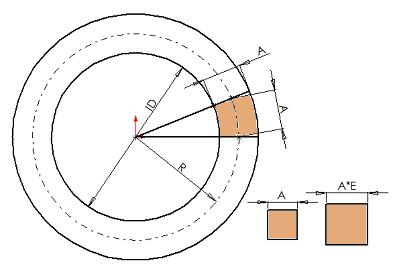## Thermal Expansion of a Ring

Thermal expansion is a pretty easy concept to grasp.  You heat up a steel disk and its diameter increases as well as it gets thicker.  But a point of confusion arises when a hole is added in the center of the disk converting it to a ring.  Does the hole get larger or smaller when the ring is heated?

A line of thinking says the hole will get smaller because the material will expand into that void.  It’s easy to rationalize this, however it is incorrect.  Here’s an attempt at a mathmatical proof.  Please go easy on me and my assumptions/simplifications.

Without going into calculus, consider a ring cut up into 360 x 1degree segments. Now look at an individual cell along the neutral axis which has initial radius R0.  For small angles let’s assume this cell is a square.The circumference of this neutral axis is C0 = 2*pi*R0 but we can also represent it as C0 = 360*A0 because A0 is 1/360th of the circumference.  Thus,

C0 = 360*A0 = 2*pi*R0
A0 = R0*2*pi/360 [solve for A0]

The initial ID0 can be calculated in terms of A0 which is the arc length and the radial width of the cell.

ID0 = 2*(R0-A0/2)
ID0 = 2*(R0 – (R0*2*pi/360)/2) [sub in for A0 from earlier equation]
ID0 = 2*R0*(1 – pi/360)        [factor out R0]
ID0 = 2*R0*(360-pi)/360        [common denominator]

Now consider this cell is heated and expands in all directions.  For this example, A1 = A0 *E where E is the expansion multiplier.  Using the same equations as before…

C1 = 360*A1 = 2*pi*R1
R1 = 360*A1/(2*pi) [solve for R1]

ID1 = 2*(R1-A1/2)
ID1 = 2*(360*A1/(2*pi) – A1/2) [sub in for R1]
ID1 = 2*A1*(360/(2*pi) – 1/2)  [factor out A1]
ID1 = 2*A1*(360-pi)/(2*pi)     [common denominator]
ID1 = 2*A0*E*(360-pi)/(2*pi)   [sub in for A1]
ID1 = 2*R0*E*(2*pi)/360*(360-pi)/(2*pi) [sub in for A0]
ID1 = 2*R0*E*(360-pi)/360      [cancel (2*pi)]

Now assume you chopped the ring into an infinite number of segments.  The equations now become:
ID0 = 2*R0*(infinity-pi)/infinity
ID1 = 2*R0*E*(infinity-pi)/infinity

(infinity-pi)/infinity is equal to infinity/infinity which equals 1 so the equations now reduce to
ID0 = 2*R0
ID1 = 2*R0*E
This makes perfect since because as the cell size gets smaller, 2*R0 is a very good estimation of the ID.

Now the big question … which is bigger – ID0 or ID1?  Take a look at these two equations and you’ll see that ID1 is larger than ID0 by a factor of E.  Surprise!